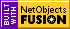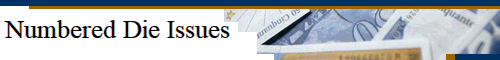Introduction. As is well known certain Roman Republican denarii of the early first century B.C. were struck with uniquely numbered dies, so that we have a good idea of how many dies were used for these issues. This gives us an opportunity for checking the predictions of formulae for estimating die numbers against reality. For some time I have therefore been collecting as many images as I can find of the examples of these types that appear in the market, and then using the resulting statistics to estimate the die numbers, using various formulae. Three separate estimates of the number of dies were used (see "Estimating Die Numbers" for details - n and d are number of coins and different dies in the sample, while dr is the number of dies appearing r times in the sample): 1)     De = nd/(n-d) This formula assumes an exponential die frequency distribution of the coins in the sample, and hence a constant rate of breakage of the dies. It is a simplified version of the formula obtained assuming a Negative Binomial die frequency distribution with a shape parameter of 1 for the sample.* 2)     D2 = d(1+d1/2d)/(1-d1/n) This assumes a Negative Binomial die frequency distribution for the sample with a shape parameter of 2, which in turn implies a somewhat truncated die lifetime distribution, such as would result if non-breaking dies were retired after a certain usage.** (2 is simply a convenient value for the shape parameter here - higher values could also be used, but in practice most samples seem to involve shape parameter values in the range of 1 to 2). 3)     Dte = d + d0 = d + d12/d2 This is the "truncated exponential" estimate, which is based a simple heuristic extrapolation of d0 (the number of dies not present in the sample) from the first two terms (d1 and d2) in the actual die frequency distribution, assuming an asymptotically exponential frequency distribution. For actual samples Dte tends to approximate De for quasi-exponential die frequency distributions (with p values around 1) but gives results closer to D2 for samples with higher p values. The results to date are summarised below. (Error ranges in the individual calculated estimates of D are not given but they will be of the order of +/- 40-50% for a 95% coverage). The nominal, or expected, die numbers have been augmented to include known official looking duplicate numbers. Also given below are the values of the die frequency distribution shape parameter p which gives the best fit of D to the expected value for each type (using Eqn 2 above with the factor 2d replaced by pd, as in Eqn 5 in "Estimating Die Numbers"). Results. Cr. 357/1  C. Norbanus: Nominal obverse dies (both reverses) 259 max (250 max excluding #220-228). n = 282, d = 137, d1 = 65, d2 = 35; R = n/d = 2.06 De: 266;   D2: 221;  Dte: 258.  p = 1.2 (250 dies), 1.0 (259 dies) Cr. 361/1  P. Crepusius: Nominal reverse dies 522. n = 220, d = 166, d1 = 122, d2 = 36; R = n/d = 1.33 De: 676;   D2: 509;  Dte: 579. p = 1.8 Cr. 378/1  C. Marius Capito: Nominal obverse and reverse dies 151. n = 163, d = 82, d1 = 38, d2 = 23; R = n/d = 1.96 De: 169;   D2: 133;  Dte: 146. p = 1.2 Cr. 382/1  C. Naevius Balbus: Nominal reverse dies 240 (+ maybe a couple more dups, say 242?). n = 207, d = 131, d1 = 77, d2 = 40; R = n/d = 1.58 De: 357;   D2: 270;  Dte: 279. p = 3.7 Cr. 383/1  Ti. Claudius Nero (ordinary series): Nominal reverse dies 152? ("170 max"). n = 112, d = 64, d1 = 36, d2 = 14; R = n/d = 1.75 De: 149;   D2: 121;  Dte: 157. p = 0.9 (152 Dies), 0.7 (170 Dies) Cr. 383/1  Ti. Claudius Nero (A series):  Nominal reverse dies 133. n = 118, d = 72, d1 = 37, d2 = 27; R = n/d = 1.64 De: 185;   D2: 132;  Dte: 123. p = 1.9 Discussion. Although the values of the sample size factor R = n/d are only moderate at most, the basic sample coverages (d/D) are mostly about 0.5 or more, high enough to give useful results. Turning to the individual figures, it will be seen that De normally gives the highest estimate for D, and in most cases overestimates D as compared with the known value. D2 underestimates D in all cases except one (Naevius), although usually by not too much, and if anything gives a better fit than De overall. The Dte estimates were above the expected value in three cases, below in one and about equal in two. They mostly split the other two estimates and overall produced the "best" estimates of the three formula. It will also be seen that as expected the Dte estimate tends toward De for low p values and D2 for higher p values, so that it effectively selects the more appropriate p value. Alternatively, choosing the middle of the three values rather than any particular estimator has something to recommend it, and in fact turns out to give estimates as good as Dte by itself, as where the middle value differs from Dte it produces better results, although only slightly. One other noticeable result is the range of p values giving the best fit to the expected values; mostly these lie in the general range of p = 1 to 2, although in the odd case of Naevius the optimal shape parameter is quite large, so that the frequency distribution tends toward a simple Binomial distribution, which, in principle at least, indicates more or less equal die outputs (although in this case analysis also shows that the sample is clearly a long way from random). So there you have it - choose your formula, or better, use all three.   *  The fuller Negative Binomial formula for the exponential case (Eqn 7 in "Estimating Die Numbers") is: D1 = d(1+d1/d)/(1-d1/n) This includes d1 and hence will give an estimate for D similar to but generally somewhat different from De. ** Alternatively, you can use this formula from Esty: D2(R) = 2n/[R-4+sqrt(8R+R2)] which uses only n and d and gives a similar but different estimate for D2.   Ross Glanfield January 2013. Latest revisions: 15 Jan. '13:   Nomenclature changed to distinguish De from D1 (and D2 from D2(R)).   13 Nov. '14: Figures updated, d1 and d2 figures added.[Home] [Articles] [Notes] [Data]